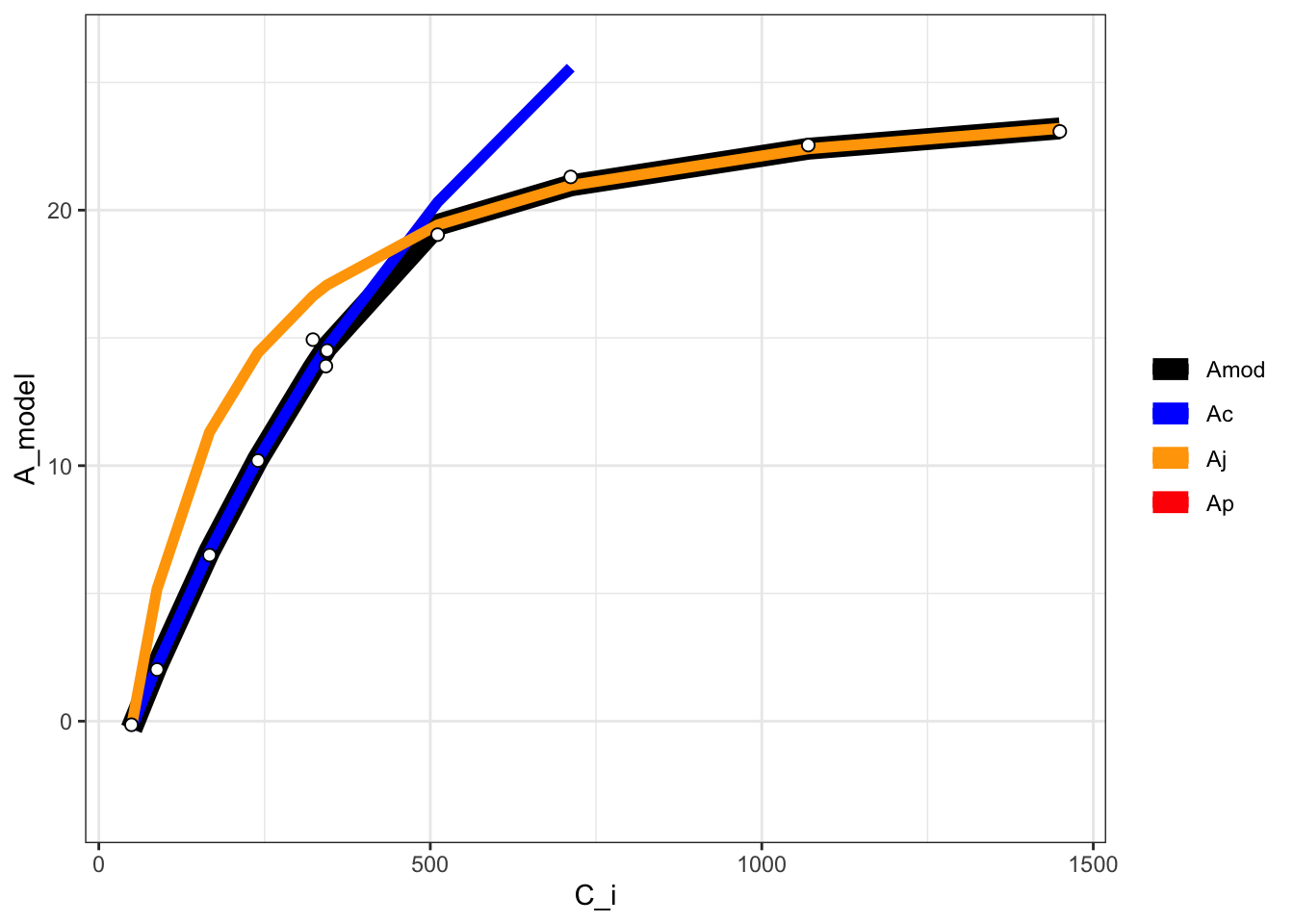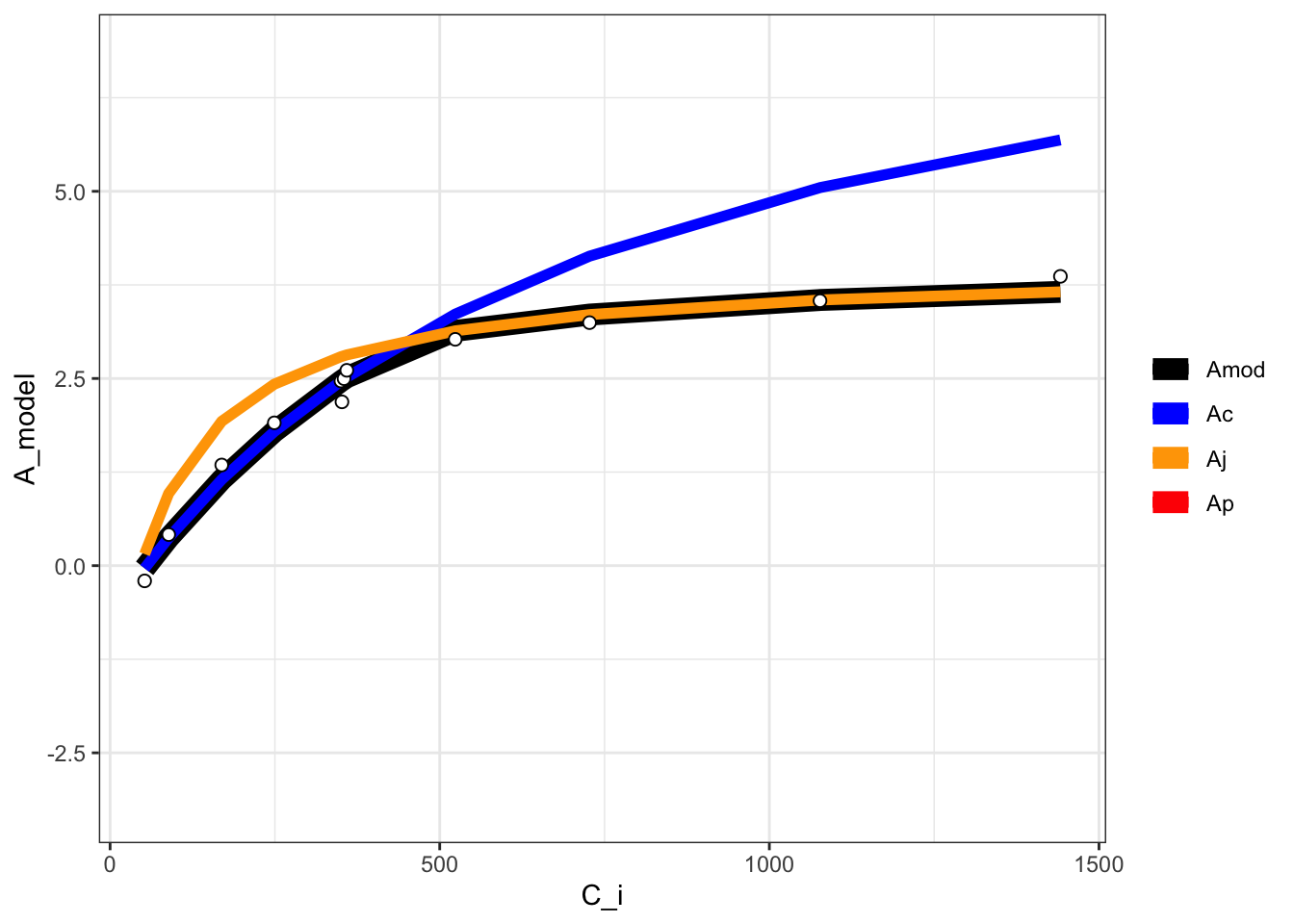This package currently implements a Gu-type fitting procedure for CO2 response curves similar to the Duursma (2015) implementation. There is ongoing work to implement a full Gu-type method whereby mesophyll conductance, Km, and GammaStar could all be fit (Gu et al. 2010).

library(dplyr)
library(photosynthesis)

dat = system.file("extdata", "A_Ci_Q_data_1.csv", package = "photosynthesis") |>
mutate(
# Set grouping variable
group = as.factor(round(Qin, digits = 0)),
# Convert data temperature to K
T_leaf = Tleaf + 273.15
) |>
rename(A_net = A, PPFD = Qin, C_i = Ci)

# Fit ACi curve.
# Note that we are filtering the data.frame to fit for a single light value
fit = fit_aci_response(filter(dat, group == 1500))

# View fitted parameters
fit[]
##   Num V_cmax V_cmax_se    J_max        J      J_se V_TPU V_TPU_se        R_d
## 6   0 62.797  2.176227 110.3051 103.9718 0.1847135  1000       NA -0.3470509
##      R_d_se     cost citransition1 citransition2 V_cmax_pts J_max_pts V_TPU_pts
## 6 0.3947545 1.063979      427.6839      1450.485          8         4         0
##   alpha alpha_g gamma_star25 Ea_gamma_star K_M25   Ea_K_M  g_mc25 Ea_g_mc Oconc
## 6  0.24       0        42.75         37830 718.4 65508.28 0.08701       0    21
##   theta_J
## 6    0.85
# View graph
fit[]
## Warning: Removed 2 rows containing missing values (geom_line()).
## Warning: Removed 12 rows containing missing values (geom_line()).# View data with modeled parameters attached
# fit[]

# Fit many curves
fits = fit_many(
data = dat,
funct = fit_aci_response,
group = "group",
progress = FALSE
)
## Warning: fit_many() was deprecated in photosynthesis 2.1.3.
## This warning is displayed once every 8 hours.
## Call lifecycle::last_lifecycle_warnings() to see where this warning was
## generated.
# Print the parameters
# First set of double parentheses selects an individual group value
# Second set selects an element of the sublist
fits[][]
##   Num  V_cmax V_cmax_se    J_max        J       J_se V_TPU V_TPU_se        R_d
## 6   0 8.94862 0.5509706 47.01527 16.63315 0.08692268  1000       NA -0.1565895
##      R_d_se      cost citransition1 citransition2 V_cmax_pts J_max_pts
## 6 0.1264438 0.1194886      441.2967      1442.493          8         4
##   V_TPU_pts alpha alpha_g gamma_star25 Ea_gamma_star K_M25   Ea_K_M  g_mc25
## 6         0  0.24       0        42.75         37830 718.4 65508.28 0.08701
##   Ea_g_mc Oconc theta_J
## 6       0    21    0.85
# Print the graph
fits[][]
## Warning: Removed 12 rows containing missing values (geom_line()).# Compile graphs into a list for plotting
fits_graphs = compile_data(fits, list_element = 2)

# Print graphs to jpeg
# print_graphs(data = fits_graphs, path = tempdir(), output_type = "jpeg")

# Compile parameters into data.frame for analysis
fits_pars = compile_data(fits, output_type = "dataframe", list_element = 1)